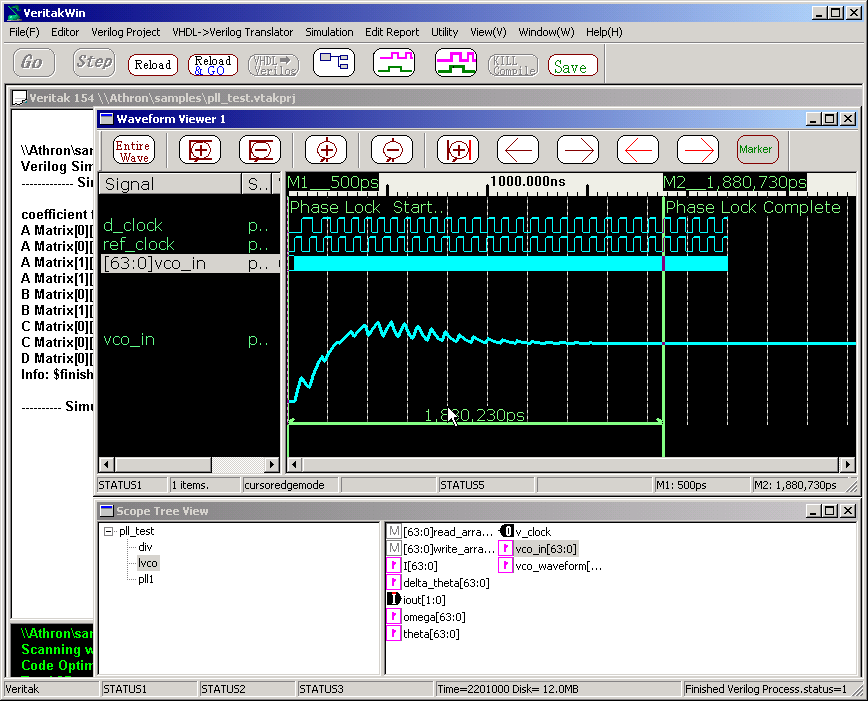2.9.1 Linear System Simulator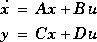State-Space model is supported.

Calculate this equation with given A/B/C/D matrix using built in 4-degree rungekutta.
Calling Procedure ;.

 \$runge_kutta("File Name",mode,Input_RealArray,Output_RealArray,[X])；
• File contains text coefficient for A/B/C/D.
• mode must be 0 at initial statement others(always) should be 1
• Give input vector u with Input_RealArray.
• Output_RealArray will get y result.
• Internal State X can be defined in HDL source as an option.

See example source of "bessel.vtakprj".
It simulates digital FM-Demudulator as below.

.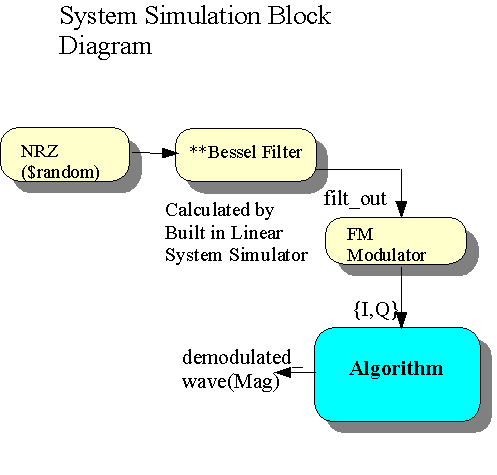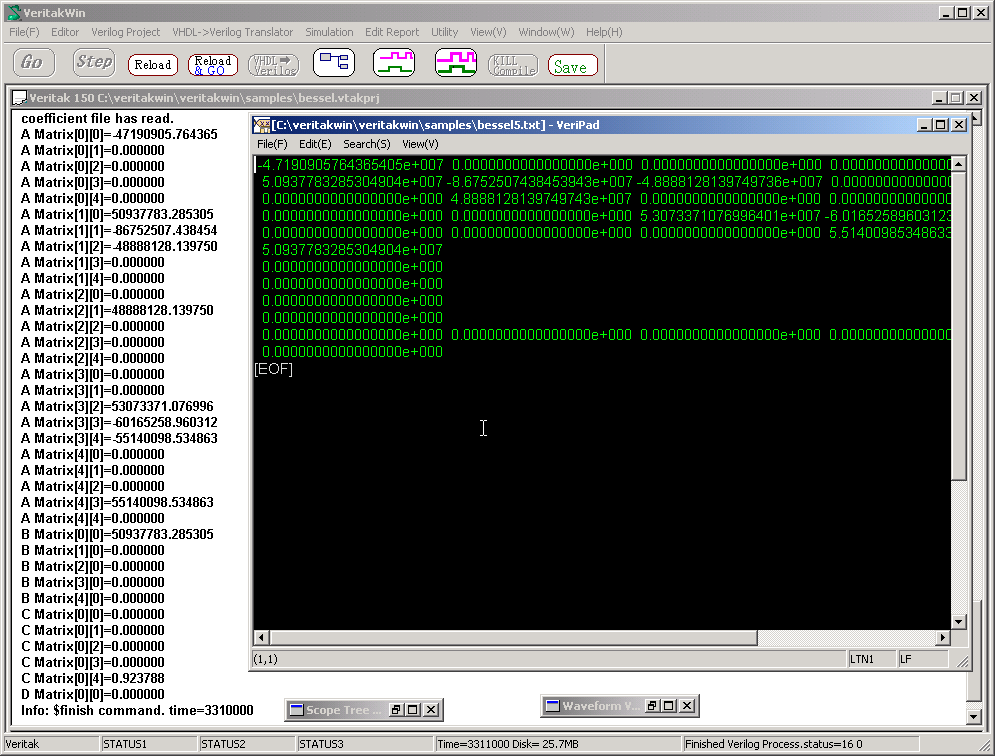Analog PLL Simulation

Fig. below shows typical analog pll block diagram.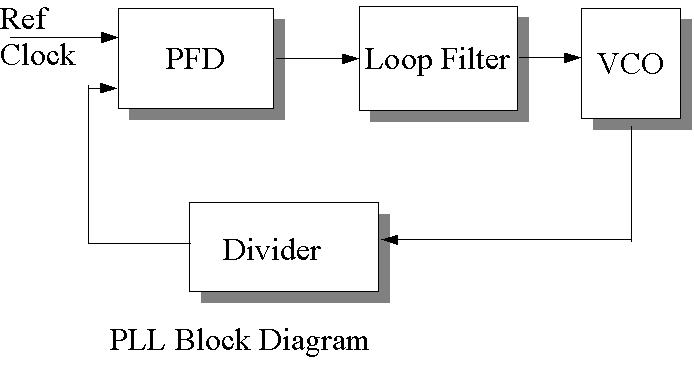Loop Filter transfer function is calculated per following equations.

Since Veritak Linear Simulator needs State-Space model, we must change the equation to State Space model. .
Matlab or Scilab can do this by tf2ss.
Following is an example.

C2=14e-12
C1=100e-12
R1=4.7e3
R1C1=R1*C1;
C1_C2=C1+C2;
C1C2R1=C1*C2*R1;
b=[R1C1 1];
a=[C1C2R1 C1_C2 0];

[A,B,C,D] = tf2ss(b,a)

freqs(b,a)
save 'F:\samples\loop_filter.txt' A B C D -ascii -double

This script generate "loop_filter.txt" in samples folder.